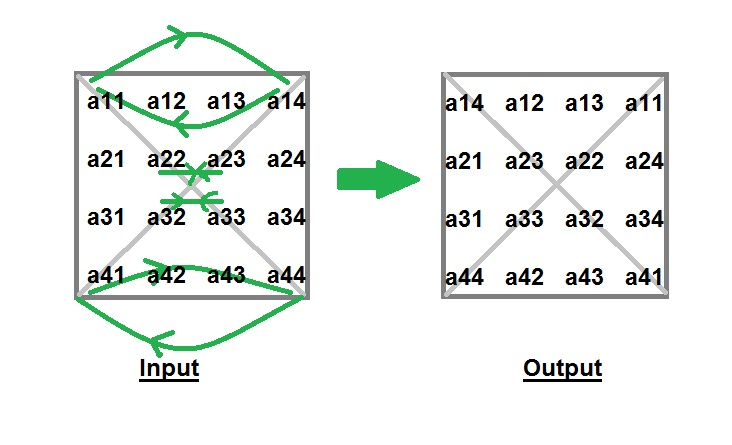Related Articles
Program to Interchange Diagonals of Matrix
• Difficulty Level : Basic
• Last Updated : 08 Jun, 2020

Given a square matrix of order n*n, you have to interchange the elements of both diagonals.

Examples :

```Input : matrix[][] = {1, 2, 3,
4, 5, 6,
7, 8, 9}
Output : matrix[][] = {3, 2, 1,
4, 5, 6,
9, 8, 7}

Input : matrix[][] = {4,  2,  3,  1,
5,  7,  6,  8,
9, 11, 10, 12,
16, 14, 15, 13}
Output : matrix[][] = {1,  2,  3,  4,
5,  6,  7,  8,
9, 10, 11, 12,
11, 14, 15, 16}
```

## Recommended: Please try your approach on {IDE} first, before moving on to the solution.

Explanation : Idea behind interchanging diagonals of a square matrix is simple. Iterate from 0 to n-1 and for each iteration you have to swap a[i][i] and a[i][n-i-1].Time complexity : O(n)

## C

 `// C program to interchange  ` `// the diagonals of matrix ` `#include ` `using` `namespace` `std; ` ` `  `#define N 3 ` ` `  `// Function to interchange diagonals ` `void` `interchangeDiagonals(``int` `array[][N]) ` `{ ` `    ``// swap elements of diagonal ` `    ``for` `(``int` `i = 0; i < N; ++i) ` `    ``if` `(i != N / 2) ` `    ``swap(array[i][i], array[i][N - i - 1]); ` ` `  `    ``for` `(``int` `i = 0; i < N; ++i) ` `    ``{ ` `    ``for` `(``int` `j = 0; j < N; ++j) ` `            ``printf``(``" %d"``, array[i][j]); ` `    ``printf``(``"\n"``); ` `    ``} ` `} ` ` `  `// Driver Code ` `int` `main() ` `{ ` `    ``int` `array[N][N] = {4, 5, 6, ` `                    ``1, 2, 3, ` `                    ``7, 8, 9}; ` `    ``interchangeDiagonals(array); ` `    ``return` `0; ` `} `

## Java

 `// Java program to interchange  ` `// the diagonals of matrix ` `import` `java.io.*; ` ` `  `class` `GFG  ` `{ ` `    ``public` `static` `int` `N = ``3``; ` `     `  `    ``// Function to interchange diagonals ` `    ``static` `void` `interchangeDiagonals(``int` `array[][]) ` `    ``{ ` `        ``// swap elements of diagonal ` `        ``for` `(``int` `i = ``0``; i < N; ++i) ` `            ``if` `(i != N / ``2``) ` `            ``{ ` `                ``int` `temp = array[i][i]; ` `                ``array[i][i] = array[i][N - i - ``1``]; ` `                ``array[i][N - i - ``1``] = temp; ` `            ``} ` ` `  `        ``for` `(``int` `i = ``0``; i < N; ++i) ` `        ``{ ` `            ``for` `(``int` `j = ``0``; j < N; ++j) ` `                ``System.out.print(array[i][j]+``" "``); ` `            ``System.out.println(); ` `        ``} ` `    ``} ` `     `  `    ``// Driver Code ` `    ``public` `static` `void` `main (String[] args)  ` `    ``{ ` `        ``int` `array[][] = { {``4``, ``5``, ``6``}, ` `                        ``{``1``, ``2``, ``3``}, ` `                        ``{``7``, ``8``, ``9``} ` `                        ``}; ` `        ``interchangeDiagonals(array); ` `    ``} ` `} ` ` `  `// This code is contributed by Pramod Kumar `

## Python3

 `# Python program to interchange  ` `# the diagonals of matrix ` `N ``=` `3``; ` ` `  `# Function to interchange diagonals ` `def` `interchangeDiagonals(array): ` ` `  `    ``# swap elements of diagonal ` `    ``for` `i ``in` `range``(N): ` `        ``if` `(i !``=` `N ``/` `2``): ` `            ``temp ``=` `array[i][i]; ` `            ``array[i][i] ``=` `array[i][N ``-` `i ``-` `1``]; ` `            ``array[i][N ``-` `i ``-` `1``] ``=` `temp; ` `         `  `    ``for` `i ``in` `range``(N): ` `        ``for` `j ``in` `range``(N): ` `            ``print``(array[i][j], end ``=` `" "``); ` `        ``print``(); ` ` `  `# Driver Code ` `if` `__name__ ``=``=` `'__main__'``: ` `    ``array ``=` `[ ``4``, ``5``, ``6` `],[ ``1``, ``2``, ``3` `],[ ``7``, ``8``, ``9` `]; ` `    ``interchangeDiagonals(array); ` `     `  `# This code is contributed by Rajput-Ji `

## C#

 `// C# program to interchange  ` `// the diagonals of matrix ` `using` `System; ` ` `  `class` `GFG  ` `{ ` `    ``public` `static` `int` `N = 3; ` `     `  `    ``// Function to interchange diagonals ` `    ``static` `void` `interchangeDiagonals(``int` `[,]array) ` `    ``{ ` `        ``// swap elements of diagonal ` `        ``for` `(``int` `i = 0; i < N; ++i) ` `            ``if` `(i != N / 2) ` `            ``{ ` `                ``int` `temp = array[i, i]; ` `                ``array[i, i] = array[i, N - i - 1]; ` `                ``array[i, N - i - 1] = temp; ` `            ``} ` ` `  `        ``for` `(``int` `i = 0; i < N; ++i) ` `        ``{ ` `            ``for` `(``int` `j = 0; j < N; ++j) ` `                ``Console.Write(array[i, j]+``" "``); ` `            ``Console.WriteLine(); ` `        ``} ` `    ``} ` `     `  `    ``// Driver Code ` `    ``public` `static` `void` `Main ()  ` `    ``{ ` `        ``int` `[,]array = { {4, 5, 6}, ` `                        ``{1, 2, 3}, ` `                        ``{7, 8, 9} ` `                        ``}; ` `        ``interchangeDiagonals(array); ` `    ``} ` `} ` ` `  `// This code is contributed by vt_m. `

Output:

```
6 5 4
1 2 3
9 8 7
```